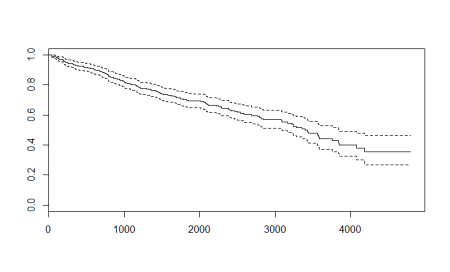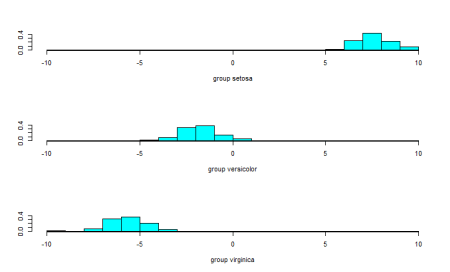Select Page

## How to implement Random Forests in R

Imagine you were to buy a car, would you just go to a store and buy the first one that you see? No, right? You usually consult few people around you, take their opinion, add your research to it and then go for the final decision. Let’s take a simpler scenario:...

## Understanding Naïve Bayes Classifier Using R

The Best Algorithms are the Simplest The field of data science has progressed from simple linear regression models to complex ensembling techniques but the most preferred models are still the simplest and most interpretable. Among them are regression, logistic, trees...

## Whys and Hows of Apply Family of Functions in R

Introduction to Looping system Imagine you were to perform a simple task, let’s say calculating sum of columns for 3X3 matrix, what do you think is the best way? Calculating it directly using traditional methods such as calculator or even pen and paper doesn’t sound...## Steps to Perform Survival Analysis in R

Another way of analysis? When there are so many tools and techniques of prediction modelling, why do we have another field known as survival analysis? As one of the most popular branch of statistics, Survival analysis is a way of prediction at various points in time....## Discriminant Analysis: Statistics All The Way

Discriminant analysis is used when the variable to be predicted is categorical in nature. This analysis requires that the way to define data points to the respective categories is known which makes it different from cluster analysis where the classification criteria...
Page 1 of 212